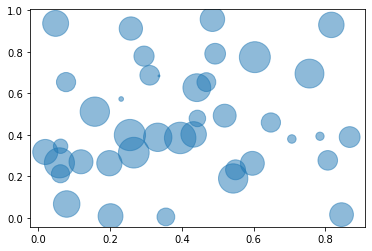A bubble plot is very similar to a scatterplot. Using matplotlib library, a bubble plot can be constructed using the `scatter()` function. In the example, the following parameters are used:

• `x` : The data position on the x axis
• `y` : The data position on the y axis
• `s` : The marker size
• `alpha` : Transparancy ratio
``````# libraries
import matplotlib.pyplot as plt
import numpy as np

# create data
x = np.random.rand(40)
y = np.random.rand(40)
z = np.random.rand(40)

# use the scatter function
plt.scatter(x, y, s=z*1000, alpha=0.5)

# show the graph
plt.show()``````## Contact & Edit

👋 This document is a work by Yan Holtz. You can contribute on github, send me a feedback on twitter or subscribe to the newsletter to know when new examples are published! 🔥